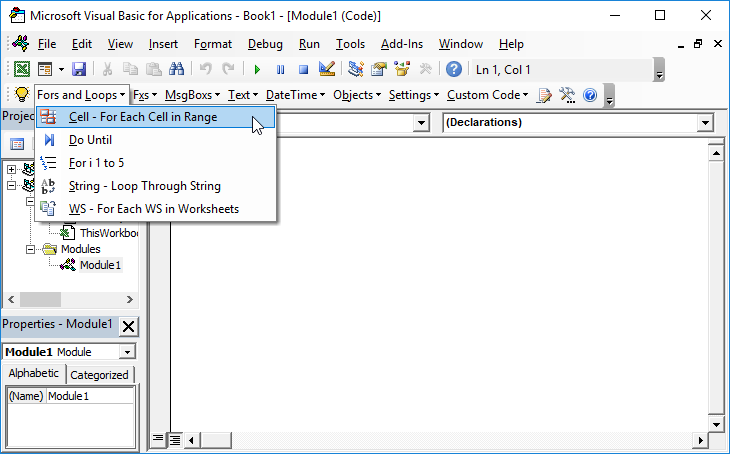# VBA – Select Every 5th Row

## Select Every 5th Row

Imagine that we have 20,000 rows of data in an Excel spreadsheet: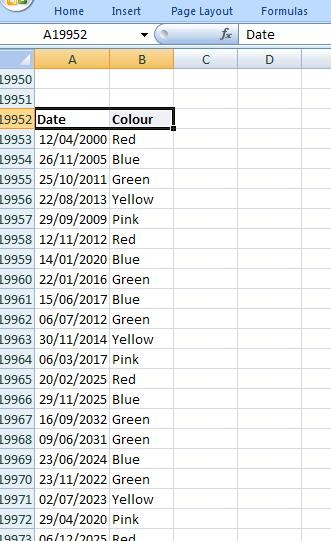However, what about if you wanted to cut this data down such as take every 5th row ? This article shows how it can be done. The Row() function gives the number of each row: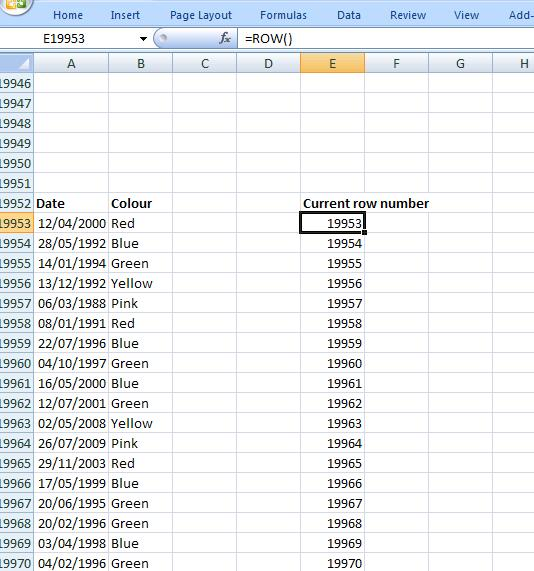``=Mod(Number,Divisor)``

Gives the remainder when “Number” is divided by “Divisor”. So for example:

``=Mod(28,5)``

Will give 3 the remainder when 28 is divided by 5.
So we can look at the row number and see what happens when we perform modulo arithmetic on it:

``=IF(Mod(Row(),5)=0,1,0)``

i.e consider the remainder when the row number is divided 5. If the remainder is 0 then put a 1 in the cell otherwise put a zero. For convenience I have hardcoded the row numbers in the above table . Now we insert additional column to work out when this happens. The formulae will be the modula formulae as above: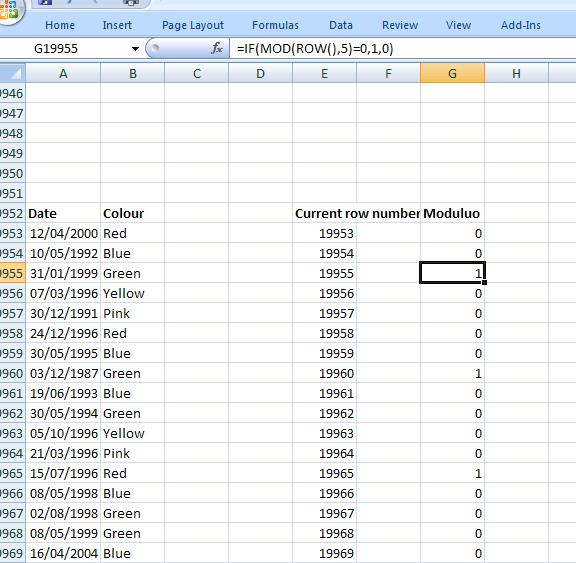We see that rows that have row numbers that are divisible by 5 have modulo 1. If we now FILTER the data to show only the 1’s: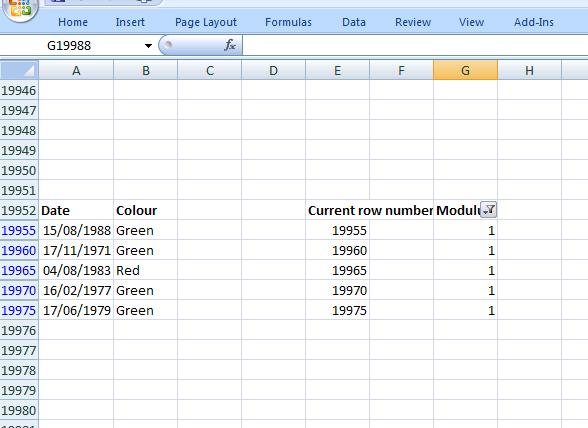If we now select Visible Cells only and then copy this data only, we have our subset of our data.

Stop searching for VBA code online. Learn more about AutoMacro – A VBA Code Builder that allows beginners to code procedures from scratch with minimal coding knowledge and with many time-saving features for all users!